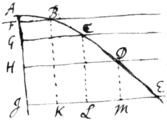<37r>

Sir

I thank you for your kind present. Mr Andersons book is very ingenious, & may prove as usefull if his principles be true. But I suspect one of them, namely that the bullet moves in a Parabola. This would be so indeed were the horizontal celerity of the bullet uniform, but I should think its motion decays considerably in the flight. Suppose for instance a bullet shot horizontally from A moves in theline AE, & AI being perpendicular to the horizon in it take AF
AG, AH, AI, &c in proportion as the square numbers 1, 4, 9, 16 &c: & its certain that if in one moment of time the bullet descend as low as F, in the next moment it shall descend as low as G, in the 3d as low as H &c. And therefore drawing the horizontall lines FB, GC, HD, IE; the bullet at the end of the first moment will be somewhere in the line FB suppose at B, & at the end of the 2d moment it will be somewhere in the line GC suppose at C &c. But that FB, GC, HD & IE are in Arithmeticall progression (which is the condition of the Parabola) seems not probable; for if it were so, the celerity of the bullet would increas becaus the spaces AB, BC, CD, DE described in equall times are the latter bigger than the former: whereas I should rather think that the celerity decreases very considerably. And perhaps this rule for its decreasing may pretty nearly approach the truth, viz: Letting fall the perpendiculars BK, CL, DM &c to make IK, KL, LM &c, a decreasing Geometricall progression. If you should have occasion to speak of this to the Author, I desire you would not mention me becaus I have no mind to concern my self further about it.

As for the method of extracting the roots of literal equations, the root of this ${y}^{3}+aay-{b}^{3}=0$ (which may be a form for all cubic equations) may be thus extracted. Suppose l pretty nearly equal to the desired root & put l in the quotient for the first term. Then proceed as follows.

$\begin{array}{l}\begin{array}{cc}\begin{array}{cc}\begin{array}{cc}\phantom{________________}& \phantom{__________}\end{array}& \left(l-\frac{m}{n}-\frac{3lmm}{{n}^{3}}+\frac{{m}^{3}}{{n}^{4}}\right\\ \phantom{.}\end{array}& \end{array}\\ \begin{array}{cc}\begin{array}{c}\phantom{.}\\ l+p=y& {y}^{3}& \phantom{\rule{0.2em}{0ex}}{l}^{3}+3llp+3lpp+{p}^{3}\phantom{\rule{0.5em}{0ex}}\text{.}\hfill \\ & +aay& \phantom{\rule{0.2em}{0ex}}+{a}^{2}l+aap\phantom{\rule{0.5em}{0ex}}\text{.}\hfill \\ & -{b}^{3}& -{b}^{3}\phantom{\rule{0.5em}{0ex}}\text{.}\hfill \\ \phantom{________________}& \phantom{__________}\end{array}& \begin{array}{l}\text{For brevities sake I have put}\phantom{\rule{0.5em}{0ex}}{l}^{3}+aal-{b}^{3}=m\\ \text{&}\phantom{\rule{1em}{0ex}}3ll+aa=n\phantom{\rule{0.5em}{0ex}}\text{.}\end{array}\end{array}\\ \begin{array}{cc}\begin{array}{c}\phantom{.}\\ -\frac{m}{n}+q=p& {p}^{3}& -\frac{{m}^{3}}{{n}^{3}}+\frac{3mmq}{nn}-\frac{3mqq}{n}+{q}^{3}\phantom{\rule{0.5em}{0ex}}\text{.}\hfill \\ & +3lpp& \phantom{\rule{0.2em}{0ex}}+\frac{3lmm}{nn}+\frac{6lm}{n}q+3lqq\phantom{\rule{0.5em}{0ex}}\text{.}\hfill \\ & +np& -m+nq\phantom{\rule{0.5em}{0ex}}\text{.}\hfill \\ & +m& \phantom{\rule{0.2em}{0ex}}+m\phantom{\rule{0.5em}{0ex}}\text{.}\hfill \\ \phantom{________________}& \phantom{__________}\end{array}& \end{array}\\ \begin{array}{cc}\begin{array}{c}\phantom{.}\\ -\frac{3lmm}{{n}^{3}}+r=q& {q}^{3}& -\frac{27{l}^{3}{m}^{6}}{{n}^{9}}+\frac{27ll{m}^{4}}{{n}^{6}}r-\frac{9lmm}{{n}^{3}}rr+{r}^{3}\phantom{\rule{0.5em}{0ex}}\text{.}\hfill \\ & -\frac{3m}{n}qq& -\frac{27ll{m}^{5}}{{n}^{7}}+\frac{18l{m}^{3}}{{n}^{4}}r-\frac{3m}{n}rr\hfill \\ & +3lqq& \phantom{\rule{0.2em}{0ex}}+\frac{27{l}^{3}{m}^{4}}{{n}^{6}}-\frac{18llmm}{{n}^{3}}r+3lrr\hfill \\ & +\frac{3mm}{nn}q& -\frac{9l{m}^{4}}{{n}^{5}}+\frac{3mm}{nn}r\hfill \\ & -\frac{6lm}{n}q& \phantom{\rule{0.2em}{0ex}}+\frac{18ll{m}^{3}}{{n}^{4}}-\frac{6lm}{n}r\hfill \\ & +nq& -\frac{3lmm}{nn}+nr\hfill \\ & -\frac{{m}^{3}}{{n}^{3}}& -\frac{{m}^{3}}{{n}^{3}}\phantom{\rule{0.5em}{0ex}}\text{.}\hfill \\ & +\frac{3lmm}{nn}& \phantom{\rule{0.2em}{0ex}}+\frac{3lmm}{nn}\phantom{\rule{0.5em}{0ex}}\text{.}\hfill \\ \phantom{________________}& \phantom{__________}\end{array}& \end{array}\end{array}$

<37v>

There may be other ways of extracting the root of this equation: as for instance if b be greater then a, the root may be thus exprest $y={b}^{3}-\frac{aa}{3b}+\frac{{a}^{6}}{81{b}^{5}}$$+\frac{{a}^{8}}{243{b}^{7}}\phantom{\rule{0.5em}{0ex}}\text{&c}$. Or thus $y=\frac{{b}^{3}}{aa}-\frac{{b}^{9}}{{a}^{8}}+\frac{3{b}^{15}}{{a}^{14}}-\frac{12{b}^{21}}{{a}^{20}}\phantom{\rule{0.5em}{0ex}}\text{&c}$ if a be considerably greater then b.

The extraction of the simple cube root may be done after the same manner omitting only the 2d terme $aay$. Or els it may be done as in numerall Arithmetic. Suppose ${y}^{3}={a}^{3}+{b}^{3}$, & that a is bigger then b, & let the divisor for finding the terms of the Quotient be always triple the square of the first term, that is $3aa$. And the form of the work will be this.

$\begin{array}{l}\begin{array}{lllll}{a}^{3}& +& {b}^{3}& \phantom{\rule{0.5em}{0ex}}& \left(a+\frac{{b}^{3}}{3aa}-\frac{{b}^{6}}{9{a}^{5}}+\frac{5{b}^{9}}{81{a}^{8}}\phantom{\rule{0.5em}{0ex}}\text{&c}\right\end{array}\\ \begin{array}{lll}{a}^{3}& & \\ 0& +& {b}^{3}\end{array}\\ \begin{array}{lll}\phantom{{0}^{0}}& \phantom{+}& \begin{array}{lllll}{b}^{3}& +& \frac{{b}^{6}}{3{a}^{3}}& +& \frac{{b}^{9}}{27{a}^{6}}\\ 0& -& \frac{{b}^{6}}{3{a}^{3}}& -& \frac{{b}^{9}}{27{a}^{6}}\end{array}\end{array}\\ \begin{array}{llll}\phantom{{0}^{0}}& \phantom{+}& \phantom{{0}^{0}}& \begin{array}{llllllllll}-& \frac{{b}^{6}}{3{a}^{3}}& -& \frac{2{b}^{9}}{9{a}^{6}}& \phantom{\rule{0.5em}{0ex}}& \ast & +& \frac{{b}^{15}}{81{a}^{12}}& +& \frac{{b}^{18}}{729{a}^{15}}\\ & \hfill 0\hfill & -& \frac{5{b}^{9}}{27{a}^{6}}& \phantom{\rule{0.5em}{0ex}}& \ast & -& \frac{{b}^{15}}{81{a}^{12}}& -& \frac{{b}^{18}}{729{a}^{15}}\end{array}\end{array}\end{array}$

So if ${y}^{3}=c$, suppose any letter as a to be pretty nearly equall to the cube root of c, & putting $c-{a}^{3}=b$ or ${a}^{3}+b=c$ extract the cube root out of ${a}^{3}+b$ as in the former example; which root $\left(\text{viz:}\phantom{\rule{0.5em}{0ex}}a+\frac{{b}^{3}}{3aa}-\frac{{b}^{6}}{9{a}^{5}}\right$$+\frac{5{b}^{9}}{81{a}^{8}}\phantom{\rule{0.5em}{0ex}}\text{&c})$ being once extracted may be kept as a rule for extracting numeral cube roots. But yet (as you suggest) these infinite series are only usefull where the roots of equations cannot be attained accurately. For when they can be accurately attaind recours must be had to other methods, this only performing it by approximation.

Sir I am

Is. Newton

Cambridge. June 20.
1674.

<37av>

These

For Mr John Collins at the
Farthing Office in Fenchurch
Street in

London.

2

Parabola and extracting of
rootes in Species